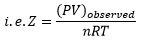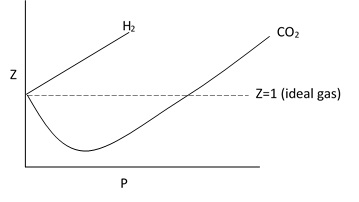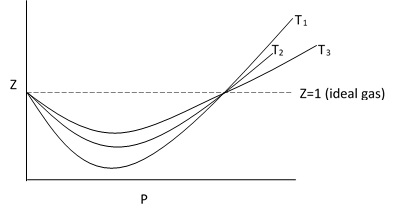## TyroCity

Chemistry 11 Notes for Chemistry Notes

Posted on • Updated on

# Explanation Of Gas Laws Based On Kinetic Theory

Boyle’s law:
The pressure of the gas is due to the collision of gas molecules on the wall of the container. The pressure depends on:

1. The number of molecules striking the wall per unit interval of time.
2. The force with which the molecules strike the wall.

If the volume is reduced to half, the number of the molecules colliding on the wall will be doubled. Hence, pressure will also be doubled. This is the Boyle’s Law.

Charle’s law:
If the temperature of a gas inside a container is increased the pressure will increase due to increased collision of the molecules on the wall of the container. However, if the pressure is kept constant, the volume must increase in order to avoid the increase in molecular collision.

Deviation from ideal behavior:
Ideal gases are those gases for which the gas laws are applicable under all conditions of temperature and pressure.
For an ideal gas, the equation PV = nRT is true at all temperature However none of the gas are ideal. The gases which doesn’t obey ideal gas equation at all temperature and pressure are known as real gases.

For a real gas, PV ≠ nRT.
The ratio of the product of pressure and volume to nRT is known as compressibility factor (Z).So, for a ideal gas the volume of Z is 1. For real gas the value of Z is less or greater than 1.

The deviation from ideal behavior shown graphically by plotting a graph between Z and P.The deviation from ideal gas behavior decreases with increase in temperature.ig: plot of Z vs P for a single gas at different temperature.

Cause of deviation:
The cause of deviation of real gases from ideal behavior was first explained by Van der Waal. He suggested the cause of deviation is mainly due to two faulty assumptions made in the kinetic theory of gases. They are:

1. The force of interaction between gas molecules is negligible.
2. The volume of gas molecules is negligible compared to the total volume of the gas.

These two statements are not true at all conditions. At low temperature and high pressure, the kinetic energy of gas molecules is low and the intermolecular distance is small. So, the force between the molecules is not negligible. Similarly, the volume of gas molecules also cannot be neglected.
At high temperature and low pressure, the Kinetic energy of gas is high and intermolecular distance is also high. Therefore, the intermolecular force is negligible and the volume of gas molecules is very small compared to the total volume.
Hence, real gas exhibit ideal behavior at high temperature and low pressure.